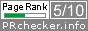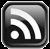# LEARN MS-ACCESS TIPS AND TRICKS

Learn Microsoft Access Advanced Programming Techniques, Tips and Tricks.

### Continued from Last Week's Post

This is the continuation of last week's Article Learn Binary Numbering System

Last week we have gone through the fundamentals of the Binary Numbering System and learned how to convert decimal number 10 to binary and seen different methods also.

I hope you have tried converting the sample number 255, that I have given at the end of last week's Article, using both methods shown there.

If you could not do it then let us do it here.

#### Method-1:

1. Find the highest Integer Value from the Binary Table that goes into the Decimal Number and subtract that value from it. Here, 128 is the highest value that can be taken.

2.  255 -128 =127

3. Write Binary digit 1 at the 128 number position underneath the Binary Table.
4.  215 214 213 212 211 210 29 28 27 26 25 24 23 22 21 20 32,768 16,384 8,192 4,096 2,048 1,024 512 256 128 64 32 16 8 4 2 1 1
5. The next highest integer number from the Binary Table that goes into 127 is 64.
6.  127 -64 =63
 215 214 213 212 211 210 29 28 27 26 25 24 23 22 21 20 32,768 16,384 8,192 4,096 2,048 1,024 512 256 128 64 32 16 8 4 2 1 1 1
7. Repeat this method till you get the value for the unit position.
 215 214 213 212 211 210 29 28 27 26 25 24 23 22 21 20 32,768 16,384 8,192 4,096 2,048 1,024 512 256 128 64 32 16 8 4 2 1 1 1 1 1 1 1 1 1

You can cross-check the result by adding up all values taken from the 1s bit (the name for the binary digit) position to arrive at the value you were trying to convert into Binary.

#### Method-2:

1. Divide the Decimal Number by 2 and take the reminder value and write at the unit position in the Binary Table.

255/2 = Quotient = 127, Remainder = 1

2. Next step, take the Quotient Value (127) of the previous calculation, divide it by 2, and find the remainder. Write the remainder value to the left of the earlier written binary digit (bit).  Repeat this process till nothing left to divide and write the final remainder value to the binary table.

127/2 = Quotient = 63,  Remainder = 1

63/2   =  Quotient = 31,  Remainder = 1

31/2   =  Quotient = 15,  Remainder = 1

15/2   =  Quotient =   7,  Remainder = 1

7/2   =  Quotient =     3,  Remainder = 1

3/2   =  Quotient =     1,  Remainder = 1

1/2   =  Quotient =     0,  Remainder = 1

You will get the Binary Number 11111111 of Decimal Number 255.

You can experiment with this with bigger Decimal Values or write some unknown Binary Values with random 1s and 0s and try converting them into Decimal Numbers.

Next, let us try some additions and subtractions with Binary Numbers.  If you know how to do additions and subtractions with Decimal Numbers then you will have no problems with Binary Numbers.

 11101110 238 +1110111 119 101100101 357

Start adding from the rightmost digits:

1.   0+1 = 1
2.   next 1+1 = 2, put 0 and carry 2 to the next position (like 5+5=10 we put 0 at the unit's position and carry 1 to the next position to add)
3.   next 1+1+1 carry = 3(binary 11) , put 1 and carry 2 to the next position
4.   next 1+1 carry = 2(binary 10), put 0 and carry 2 to the next position
5.   Next 1+1 carry = 2(binary 10), put 0 and carry 2 to the next position
6.   Next 1+1+1 carry = 3(binary 11), put 1 and carry 2 to the next position
7.   Next 1+1+1 carry = 3(binary 11), put 1 and carry 2 to the next position
8.   Next 1+1 carry = 2(binary 10), put 0 and put 1 to the next position.

Example: Subtraction

 11001110 206 -1111111 127 1001111 79
1.   0-1 cannot be done, so take 2 from the next position, now 2-1 = 1, but the next position on the first line becomes 0.
2.   0-1 cannot be done, so take 2 from the next position, now 2-1 = 1, next position on the first line becomes 0.
3.   0-1 cannot be done, so take 2 from the next position, now 2-1 = 1, now the next 3 positions become 0.
4.   Take Value from the 8 value position and move forward to the 4 position and to the 2 value position, 2-1 = 1
5.   1-1 = 0
6.   1-1 = 0
7.    After moving the value forward from the 7th digit position on the top line, it is now 0.  So move 2 from the next position. 2-1 = 1

You can try it out yourself, starting with smaller binary values and progressively with bigger ones.

For your information, there is no Multiplication or Division in computers.  These calculations are achieved by successive addition or successive subtraction of values.

Continued../-

Share:

#### 1 comment:

1.There are a couple of practice quizzes here. Repetition helps.

http://plentysmart.com/comp/bin1/binary1.php

Comments subject to moderation before publishing.

## TranslateSubscribe in a readerYour email address:

Delivered by FeedBurner